# Module 1: INITIAL SETUP AND BASIC OPERATION

## Learning Objectives

After reading this lesson, you should be able to:

1. choose different window layouts in MATLAB,

2. change the MATLAB user preferences.

Most of the MATLAB preferences covered in this lesson are just that. However, there are a few changes that can make a functional difference while you are learning to program, so it would be wise to familiarize yourself with the options available.

## How can I change the window layout in MATLAB?

MATLAB makes it simple to change the position and layout in the MATLAB program, and there are various ways to accomplish this.

One way is to use the layout options for each sub-window, which can be minimized, maximized, undocked, or closed by selecting the symbol in its upper right-hand corner as shown in Figure 1. Note that undocking means that the window can be separately and independently moved around from the MATLAB software (try it out!).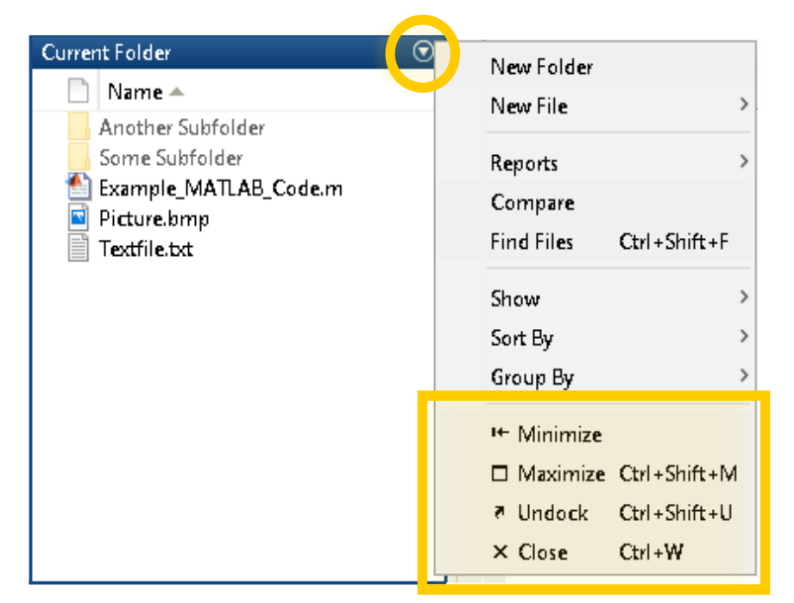Figure 1: Changing view of a window in MATLAB.

If you happen to “lose” or accidentally close a window, the simplest way to recover it is by selecting one of the MATLAB preset layout options that can be found in the “Home” navigation ribbon and then under the “Layout” button as shown in Figure 2.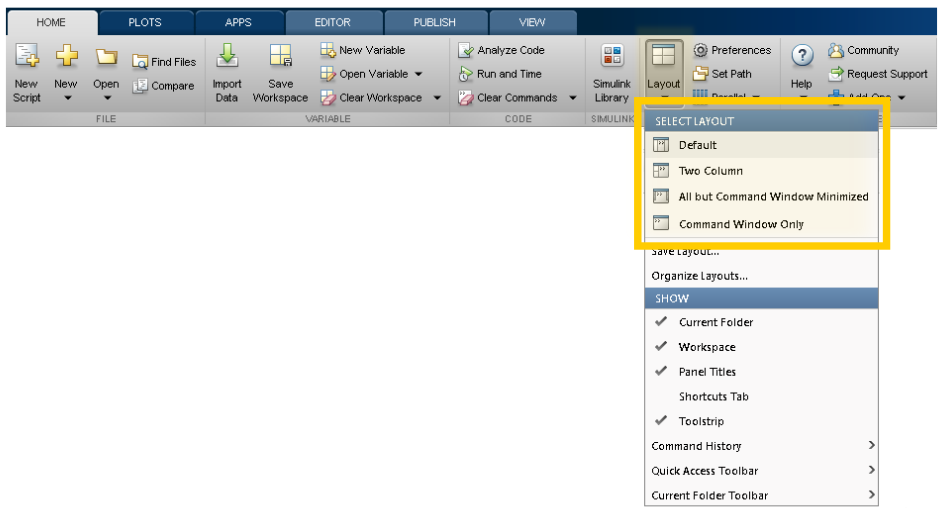Figure 2: Selecting a layout in MATLAB.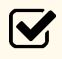Important Note: Moving forward, this course will assume that you are working with the “Default” layout preset.

## Changing Basic User Preferences

It is advantageous to change the default user preferences when working with MATLAB. For example, choose a more readable font or font size. These preferences may include visual/layout (font, font size) and functional (MATLAB behavior) options. While there are many fundamental preferences that can be changed, we will only demonstrate the font size here. Increasing the font size can make dense, small code feel more readable and less overwhelming.

Preferences can be found on the “Home” ribbon, in the “Environment” group, select the “Preferences” button as shown in Figure 3.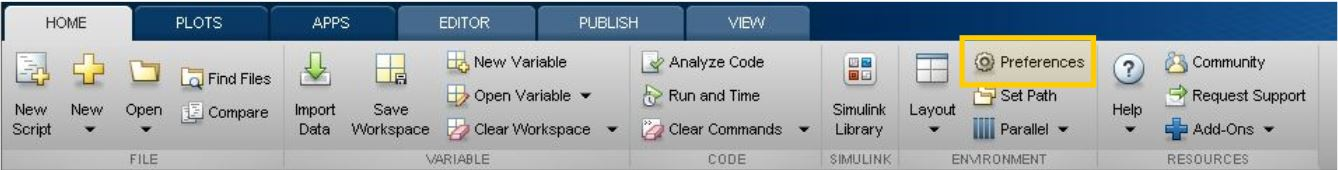Figure 3: The MATLAB preferences location on the Home tab.

Once in the Preferences pop-up window (see Figure 4), select “Fonts” in the navigation tree on the right-hand side. Then in the “Desktop code font” section, select “12” as the font size. Once done, select “OK” at the bottom of the window.

Although it is not necessary to remember exactly how to change these preferences, it is good to keep in mind that most visual aspects of MATLAB are easily changeable to suit your needs/preference.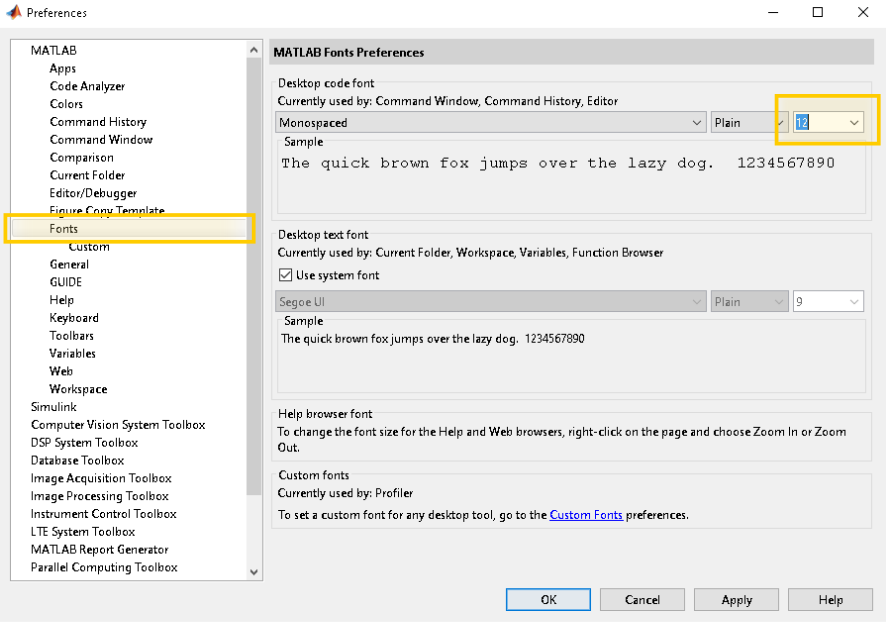Figure 4: MATLAB preferences window.